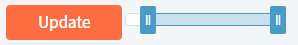# Strange behaviour of range slider

Hi,

I created a range slider that behaves a little strange. To reproduce, please create a new Blazor PWA in Visual Studio and simply add the following two lines in Index.razor:

``````<RadzenSlider Range="true" Min="-50m" Max="10m" @bind-Value=@minMax TValue="IEnumerable<int>" />
@code{ IEnumerable<int> minMax = new int[] { 0, 8 };}
``````

This results inThe left handle can be moved to the nav menu and even out of the browser window:How can I avoid this?

Many thanks

Here is another unexpected behaviour of the range slider. I only added the following code to a new Visual Studio Blazor PWA project in Index.razor:

``````<RadzenButton Text="Update" Click="UpdateSlider"></RadzenButton>
<RadzenSlider Range="true" Min="@minim" Max="@maxim" Value=@minMax TValue="IEnumerable<double>" />

@code{
decimal minim = 0m;
decimal maxim = 100;

IEnumerable<double> minMax = new double { 25d, 55d };

{
minim = 10m;
maxim = 80m;
minMax = new double { 25d, 55d };
} }
``````

After clicking the button, the left handle cannot be moved to the left minimum anymore:It stops at 12.500% (what is 10/80):

Any help is appreciated.

It is becoming even stranger. I added another slider and a click on the first one changes the second one.

``````<RadzenSlider Range="true" Min="@minim1" Max="@maxim1" @bind-Value=@minMax1 TValue="IEnumerable<double>" />
<br />
<RadzenSlider Range="true" Min="@minim" Max="@maxim" Value=@minMax TValue="IEnumerable<double>" />

@code{
decimal minim1 = 0m;
decimal maxim1 = 100m;
IEnumerable<double> minMax1 = new double { 25d, 55d };

decimal minim = 0m;
decimal maxim = 100m;
IEnumerable<double> minMax = new double { 25d, 55d };

{
minim = 10m;
maxim = 80m;
minMax = new double { 50d, 75d };
} }
``````

If you move the handles of the lower one first and afterwards the handles of the upper one, the handles of the lower one "jump" back to their original position.
How can I avoid this?

Thanks again.

At the moment negative values are not supported by RadzenSlider - we accept pull requests.

Thank you enchef.
OK, I can probably deal with positive values only by transforming my min and max values to temporary min and max values that are positive.

May I also ask for help with the other two problems:

Thanks a lot.

Hey @Flippowitsch,

Are you looking for paid support? The options are listed here: Pricing

We are already investigating the negative range issue. Please bear in mind that we guarantee replies only to Radzen Professional and Enterprise subscribers. We reply at our own discretion otherwise - mostly based on current load and priorities.

Hi @korchev and @enchev,

I have full understanding for this but at the moment, full support is too expansive for me.
If my issues are bugs you are informed now. It they are features, my posts let you know, that I expected a different behaviour. If I am doing anything wrong, I will find a solution...

Thanks anyway

We've added support for negative values:

Nice!
And it will probably find its way into a nuget update.

I just saw, that you fixed the "Left handle cannot be moved to left minimum" issue. You are really fast. Thanks!